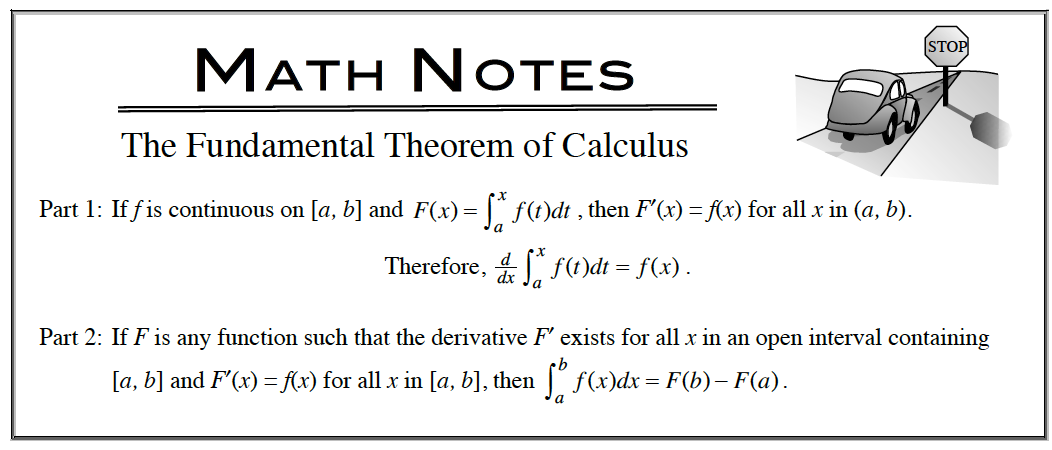### Home > APCALC > Chapter 5 > Lesson 5.1.2 > Problem5-18

5-18.

Simplify each integral expression. Homework Help ✎

1. $\int \frac { ( m ^ { 3 } - 1 ) ^ { 2 } } { m ^ { 5 } } d m$

1. Simplify the integrand by expanding the numerator and canceling out the denominator.
2. Integrate by 'undoing' the Power Rule.
3. Do not forget the + C.

1. $\int _ { 0 } ^ { 5 } ( \frac { d } { d x } \sqrt { x ^ { 2 } + 11 } ) d x$

Fundamental Theorem of Calculus (an extension):The integral of a derivative will give the original function + C.

$6-\sqrt{11}$

1. $\frac { d } { d x } [ \int _ { 0 } ^ { 5 } ( \frac { d } { d x } \sqrt { x ^ { 2 } + 11 } ) d x ]$

$=\frac{d}{dx}[\text{part (b)}]$